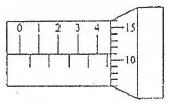# Measurement and significant figures – problems and solutions

1. The length of an object measured using a micrometer is 15.08 mm. Determine the significant figures and the number of significant figures.

A. 15.08 mm and three significant figures

B. 1.508 mm and four significant figures

C. 15.08 mm and four significant figures

D. 1.508 mm and three significant figures

Solution :

Significant figures = 15.08 mm

The number of significant figures = 4

2. A student measures the volume of a container using a small cup with a volume of 125 cm3. The container is full after filled with twenty-half cups. Determine the volume of container based on significant figure rules.

A. 2562.5 cm3

B. 2.56 x 103 cm3

C. 2563 cm3

D. 2.56 x 10-3 m3

Solution :

Volume of a cup = 125 cm3

Volume of container = 20.5 x 125 cm3 = 2562.5 cm3

3. Determine the result of measuring an object as shown in figure below, according to the rules of significant figures.

A. 4.5 mmB. 4.6 mm

C. 4.6 mm

D. 4.6 mm

Solution :

Main scale = 4.5 mm

Second scale = 11 x 0.01 mm = 0.1 mm (according to the rules of the multiplication of significant figures)

The result of measuring = 4.5 mm + 0.1 mm = 4.6 mm (according to the rules of the summing of significant figures)